# Pi Distance Keeper

ECE 5725 Spring 2022

Nivant Desai nnd25

Ben Manninen bm586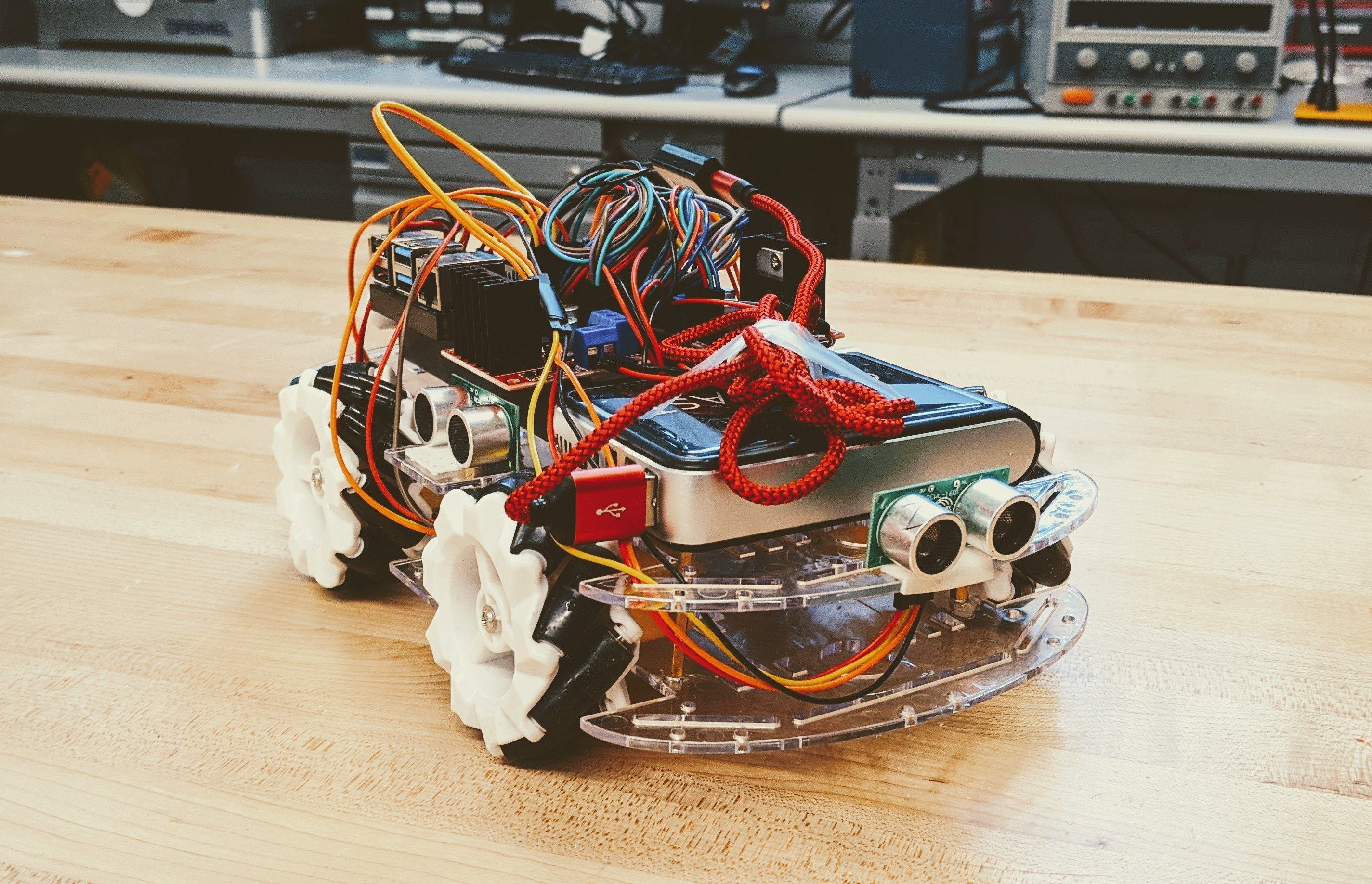## Introduction

#### Demonstration Video

This is the Distance Keeping Robot Car. The objective is for the car to be able to dodge all incoming objects that may run into it. This could be a person, another object, another car, or anything that the Robot senses around it. The Robot uses a network of four distance sensors to "see" what is going on around it. The Robot also uses omni-directional wheels to enable the traditional forward and backward motion as well as side to side movement.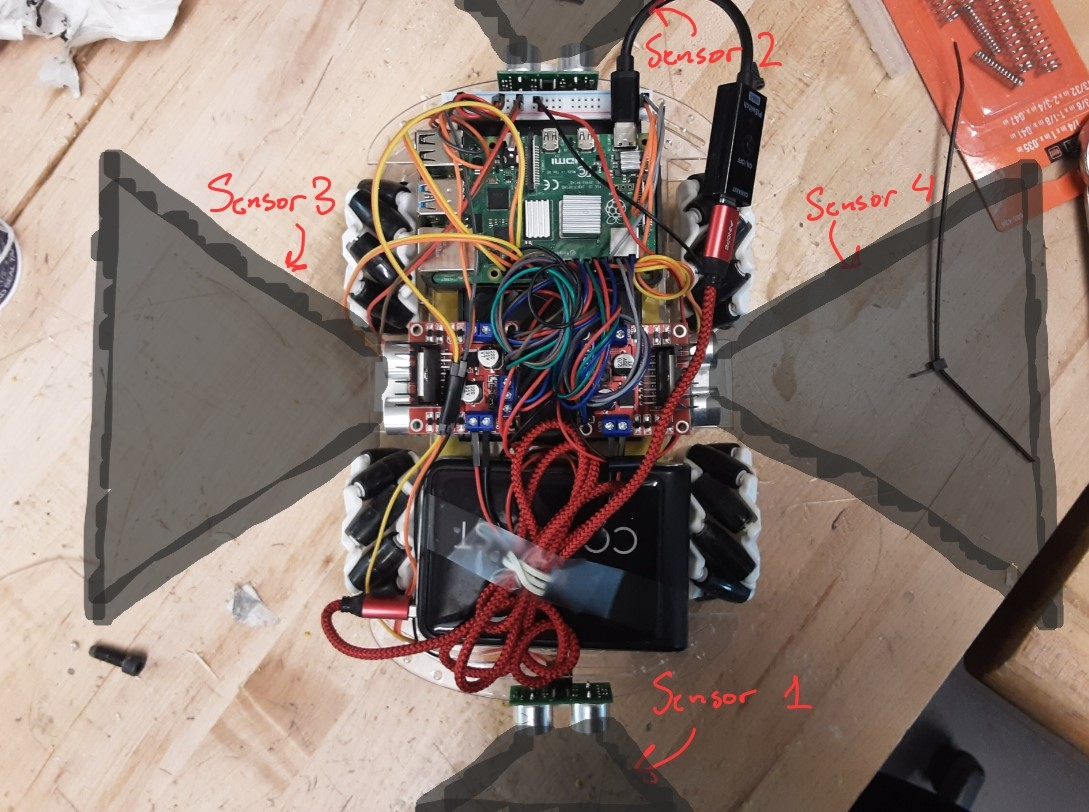Figure 1: A diagram of the  distance sensor network on the robot

As shown in Figure 1, each distance sensor has a cone shaped view that it uses to sense the distance of anything in its path. The sensors are ultrasonic and are capable of firing very quickly. There is also no worry of any sensor intruding on the view of any other since each device is mounted at a 90 degree angle with respect to the adjacent counterparts.

## Project Objectives:

• Sense the distance of any object around the Robot within a certain threshold
• Respond to objects that are within the threshold distance by moving away from them
• Handle cases when there are more than one object sensed within the threshold distance

## Design

### Hardware Design: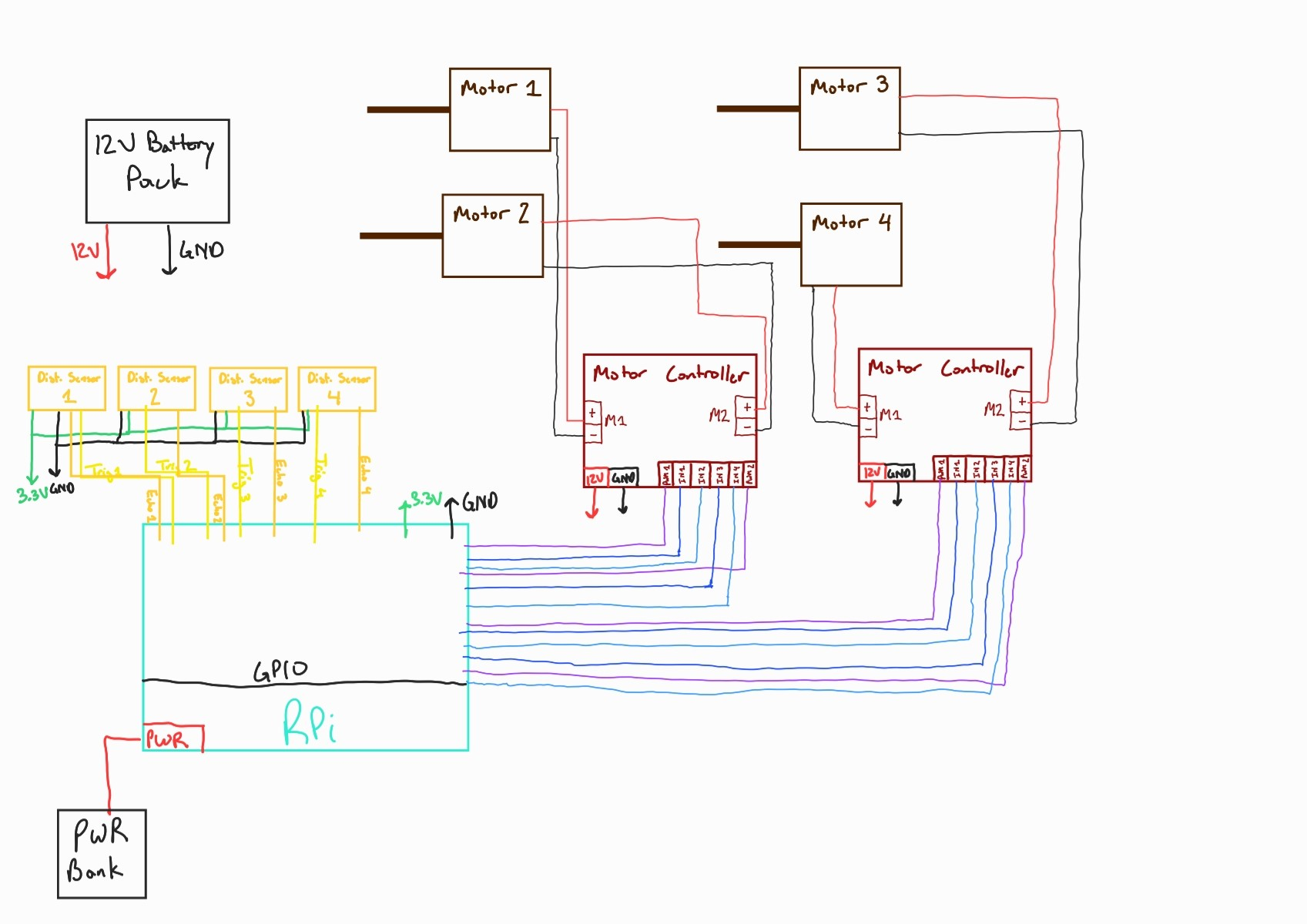Figure 2: Hardware schematic of the robot

The hardware schematic of the robot is shown in Figure 2 above. The Raspberry Pi acts as the central controller for the robot. The Pi is powered by an external power bank. It runs a python sript that allows the robot to function (discussed in the software section below). All the distance sensors connect directly to the Pi GPIO pins. Each sensor gets 3.3V and GND from the Pi. These signals are broken out on a breadboard on the robot to allow for easier wiring. Each sensor also takes in a trigger input and outputs an echo signal. These signals are integral to the actual distance sensing and are implemented in software.

There are four DC motors that need to be controlled. The robot has two L298N motor controllers which can each control two motors. The motor controllers are powered with 12V signals from a battery pack. Each controller has three signals associated with motor control for any given motor. The first is the PWM signal, taken from the Pi. The second and third are binary inputs to set the direction of the motor. A total of 12 motor control signals are wired into the Pi GPIO interface.

### Software Design

#### Multiprocessing Design: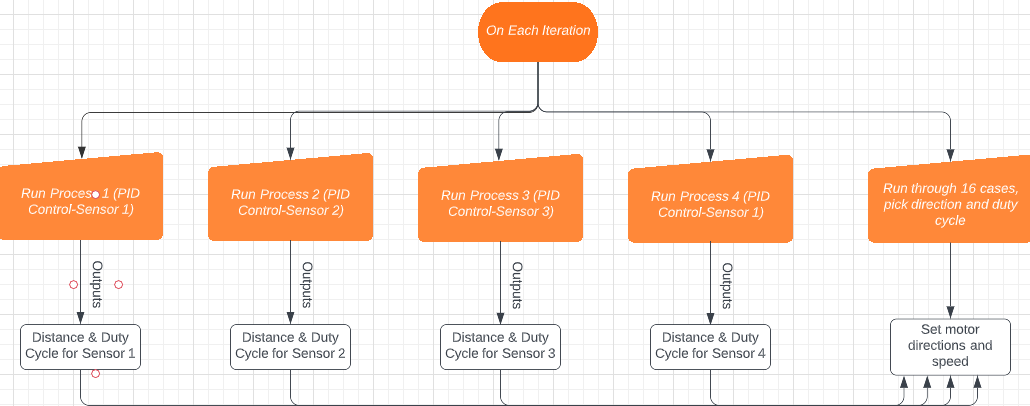Figure 3:High Level Overview of Multiprocessing Algorithm

The robot must be able to continuously check its surroundings when running. This means that each distance sensor must be operating in parallel. The only way to achieve this would be through multiprocessing. That is, each distance sensor has 1 process associated with it, and this process is continuosly writing the recorded distance and PWM signal to some shared variables. Within the mutliprocessing framework, we have 11 important shared variables. We have 4 distances for each sensor, 4 duty cycles outputed by each sensor's PID controller, the threshold distance value, a run flag for keeping processes running, and the proportional gain of the PID controller. Out of these, only the threshold distance, run flag, and proportional gain variables remain constant throughout operation. That is, these variables are only read from, not written to. The duty cycles and distances are updated on each iteration by each process respectively. The main function checks which direction the robot should be moving on any given iteration, and then sets the motor directions and duty cycles accordingly. For example, if sensor 4 has been tripped, the program sets the robot to move left, and sets all motors to the duty cycle given by sensor 4's PID controller (all other duty cycles are ignored for that iteration). The main function is explored more in the next sections.

#### PID Controller Proces: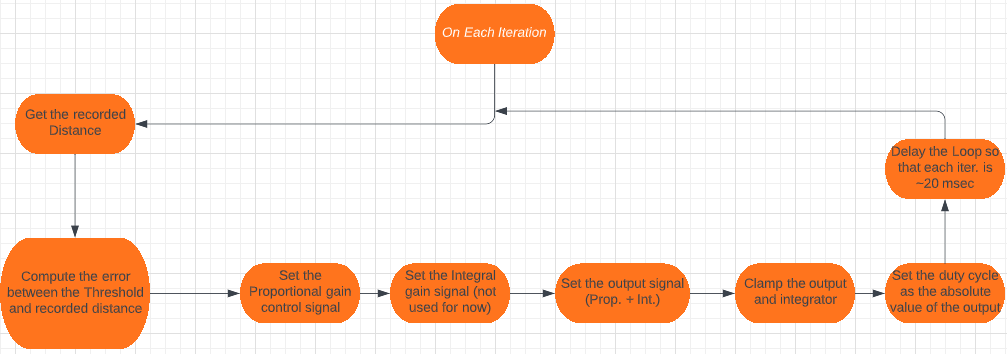Figure 4: PID Controller

Let's explore the flow of one of the sensor PID controller processes. On each iteration, we get the recorded distance of the sensor (this functionality is explored below). Based on this distance, we compute the error with the threshold value. At this point, if the error is less than zero, that means that the object is beyond the threshold, and so the duty cycle will be zero. If the error is greater than zero, then the sensor has been tripped. At this point, we set the proportional term and integral terms based on the error. The current version of code does not use the integrator, but you could choose to use it later. The output control signal is set simple as the proportional term added with the integral term. Next, we have to clamp the output and integrator. If either value is beyond 100, we simply force the value to 100. The same is true if the value is less than zero. Finally, the duty cycle is set to the absolute value of the control signal. Before ending the loop iteration, we delay the loop until 20ms has been reached. This is to make sure that each iteration takes the same amount of time. Note here that both the duty cycle and the recorded distance are shared variables.

#### Checking 16 cases for 4 sensors:

On any given iteration, we must check whether to move the robot forward, backward, left, or right. We also must set each motor's duty cycle to the appropriate value based on the sensor that has been tripped. For 4 sensors, there are 16 possible cases. For example, on any iteration, sensor 1 can be tripped and the othes not tripped. We could also have that sensor 1,2 and 4 are tripped while sensor 3 is not tripped. All of these cases are handled independently through if-statements. In the case that more than one sensor is tripped, the program will pick the sensor that has the lowest recorded distance and choose to move away from that object. The result of any of the 16 if-statements is that the motors are setup to move the robot in a certain direction (forward,backward,etc.) and the duty cycle of all motors are set to the same value.

#### Getting the distance from a distance sensor (helper function)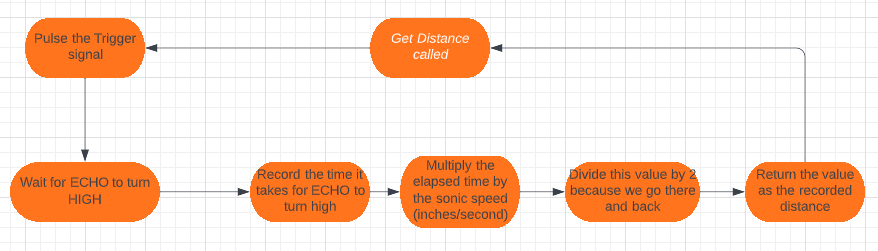Figure 5: Getting the distance from a distance sensor

How do we get the distance from an ultrasonic distance sensor? This functionality is implemented as a helper function in our code. First, we pulse the Trigger signal. That is, we set the value HIGH, wait for 1msec, and then set it back LOW. After pulsing the Trigger, we wait for the ECHO signal to output a logic 1, and record the time it takes to do so. This time is then multiplied by the sonic speed constant (which is in inches/second). Finally, we divide the value by 2 because the sonic pulse goes out and back. This value is then returned as the distance in inches.

#### Left and Right motion of the Robot: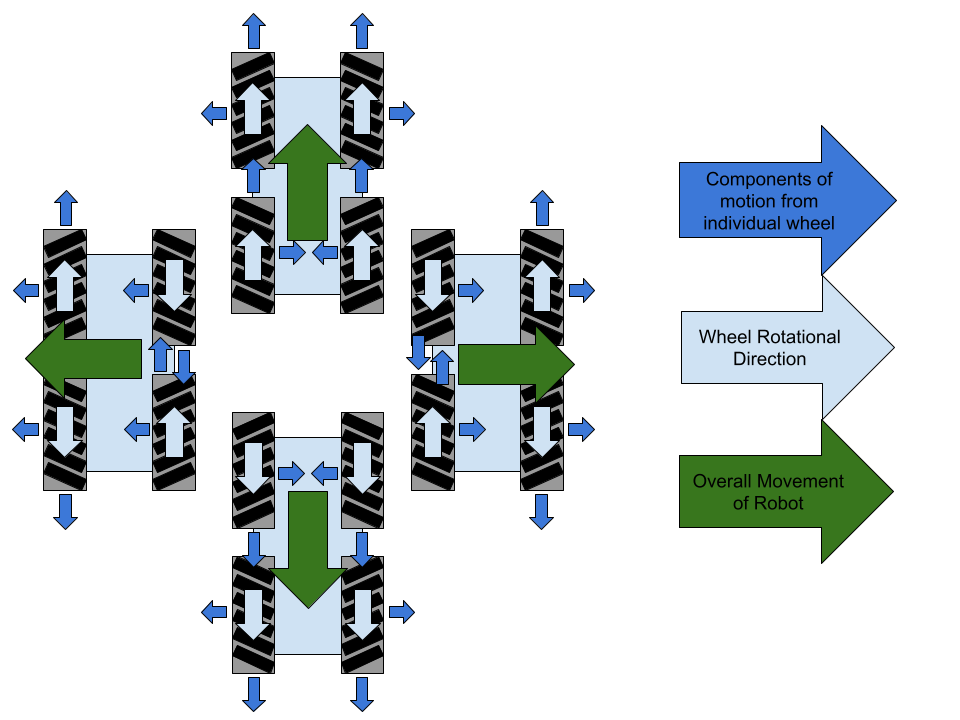Figure 6: Omni-Directional Movement

The omni-directional wheels of the robot allow us to go left and right in addition to forward and backward. How do we achieve this motion? Going forward and backward is self explanatory (simply rotate the wheels on each side clockwise or counterclockwise). If we want to go left, then the left side wheels (the wheels on the side that we are moving) must move away from each other. That is, one wheel move clockwise and the other moves counterclockwise. The other two wheels are the opposite, as they must move toward each other. All of this functionality is also implemented as helper functions (setBack, setLeft, etc.)

## Process and Testing

Our design adapted significantly over the course of this project as we encountered challenges. Initially we planned to work with a camera module to detect faces, and move the robot to faces. The first milestone for this system was to test if we could detect a face. We did not need to throughly test the accuracy of face detection, as we uesd an established model with configurable confidence levels. Once we succeeded, we quickly identified an issue: the raspberry pi was only capable of processing 1-2 frames per second. For the robot to a human's movements, this refresh rate would be significantly too slow.

We eventually able to improved the frame rate to 6-10 fps by decreasing the size of the area the model grouped as a potential face. To ensure this still fit the model, we needed to rescale the original frame.

While optimizing the face detection we also began work on using the ultrasonic distance sensors to avoid nearby objects. Given how ubiquitous ultrasonic distance sensors are, and our relatively low precision requirements, we did not test that the sensors output precise measurements. We did confirm that the output was close to correct, to validate our code. Another important test was to confirm that the sensors did not interfere with each other. Since we planned to use multiprocessing to run all four sensors simulatneously, we could not gurantee that they wouldn't fire/wait for a response at the same time. If that occured, the sensors might pick up interference from each other. We were able to confirm that they did not, likely due to being aimed in the four cardinal directions such that their sensing areas had no overlap.

For our robots movement, we checked that motors ran in the correct direction, and that the movement generated matched our expectations. Since we were working with omni-directional wheels to move holonomically, needed to confirm that we had implemented sideways motion correctly. We got this correct quickly. Movement was not as efficient as it would be with standard wheels, but that was expected due to using omni-directional wheels. Since the wheels generate force in two perpindicular directions, we force the robot to move in only one direction setting wheels to oppose each other. For our application the lost speed was not an issue.

Once we started testing the combined results, we encounted a new issue with face detection. Previously much of our testing had occured with the robot on a desk. While we did test its ability to detect faces at a distance, we did not consider the fact that there would also be a significant angle when the robot was on the floor. If someone was close to the robot, then the camera would have to be looking almost directly upward. It was unable to detect faces at this angle so we shifted to trying to detect at a distance. By aiming further away we could decrease the angle to be closer to horizontal (which is where the model was most reliable). This limited the range it could detect, and we found that due to the rescaling and cropping it was not incredibly reliable at this distance either. After trying to balance processing time against reliability, we eventually pivoted to focus on the distance sensors.

## Results and Conclusions

While we were unable to include the face detection in our final project, our distance sensor based avoidance was very reliable and reactive. The robot was able to avoid collisions even when confronted with multiple nearby objects.

Future work could explore several avenues. We could add additional sensors and allow the robot to move diagonally. This would require careful adjustment of motor pwm signals, as we would need to match the components of motion correctly, or we would move at the wrong angle. We could also attempt to implement face detection in a different manner, or choose a different object to detect with the camera. For face detection we could shift to converting images to contour maps, or other methods to bring the computational cost down. Building a mount for the camera, so it is raised above the robot closer to to head height would also make face detection easier, although depending on the height it could impact the robot's motion. Finally, we could explore completely new directions. For example, we could add a manual control system to allow us to control the robot's motion from a laptop/controller.

## Work Distribution

### Ben - bm586@cornell.edu

• Focused on debugging and optomizing the facial detection
• Implemented reactions to facial detection

### Nivant - nnd25@cornell.edu

• Initial face detection setup
• Implemented distance sensor measurements and reactions
• Setup multiprocessing

## Parts List

• Raspberry Pi 4B - \$35.00
• 2x L298N Motor Controllers - \$10
• 4x 3.3V Ultrasonic Distance Sensors - \$15.80
• 12V Battery Pack - \$8
• 8x AA battery - \$10
• Power Bank - \$25

## References

PiCamera Document
Bootstrap
Pigpio Library
R-Pi GPIO Document

## Code Appendix

``````
import time as time
import RPi.GPIO as GPIO
from multiprocessing import Queue, Value, Process
import signal as signal

#GPIO SETUP
GPIO.setmode(GPIO.BCM)
GPIO.setwarnings(False)

okSize = 200

TRIGGER_1 = 19
ECHO_1 = 26
TRIGGER_2 = 21
ECHO_2 = 20
TRIGGER_3 = 14
ECHO_3 = 15
TRIGGER_4 = 16
ECHO_4 = 12

M1_IN1 = 3
M1_IN2 = 4
M1_PWM = 2

M2_IN1 = 27
M2_IN2 = 22
M2_PWM = 17

M3_IN1 = 9
M3_IN2 = 11
M3_PWM = 10

M4_IN1 = 6
M4_IN2 = 13
M4_PWM = 5

GPIO.setup(M1_IN1, GPIO.OUT)
GPIO.setup(M1_IN2, GPIO.OUT)
GPIO.setup(M1_PWM, GPIO.OUT)

GPIO.setup(M2_IN1, GPIO.OUT)
GPIO.setup(M2_IN2, GPIO.OUT)
GPIO.setup(M2_PWM, GPIO.OUT)

GPIO.setup(M3_IN1, GPIO.OUT)
GPIO.setup(M3_IN2, GPIO.OUT)
GPIO.setup(M3_PWM, GPIO.OUT)

GPIO.setup(M4_IN1, GPIO.OUT)
GPIO.setup(M4_IN2, GPIO.OUT)
GPIO.setup(M4_PWM, GPIO.OUT)

pm1 = GPIO.PWM(M1_PWM, 1000)
pm1.start(0)
pm2 = GPIO.PWM(M2_PWM, 1000)
pm2.start(0)
pm3 = GPIO.PWM(M3_PWM, 1000)
pm3.start(0)
pm4 = GPIO.PWM(M4_PWM, 1000)
pm4.start(0)

GPIO.setup(TRIGGER_1, GPIO.OUT)
GPIO.setup(ECHO_1, GPIO.IN)
GPIO.setup(TRIGGER_2, GPIO.OUT)
GPIO.setup(ECHO_2, GPIO.IN)
GPIO.setup(TRIGGER_3, GPIO.OUT)
GPIO.setup(ECHO_3, GPIO.IN)
GPIO.setup(TRIGGER_4, GPIO.OUT)
GPIO.setup(ECHO_4, GPIO.IN)

#Sets the motors up so the robot moves left
def setLeft():
GPIO.output(M1_IN1, GPIO.HIGH)
GPIO.output(M1_IN2, GPIO.LOW)

GPIO.output(M2_IN1, GPIO.LOW)
GPIO.output(M2_IN2, GPIO.HIGH)

GPIO.output(M3_IN1, GPIO.HIGH)
GPIO.output(M3_IN2, GPIO.LOW)

GPIO.output(M4_IN1, GPIO.HIGH)
GPIO.output(M4_IN2, GPIO.LOW)

def turnLeft():
GPIO.output(M1_IN1, GPIO.HIGH)
GPIO.output(M1_IN2, GPIO.LOW)

GPIO.output(M2_IN1, GPIO.HIGH)
GPIO.output(M2_IN2, GPIO.LOW)

GPIO.output(M3_IN1, GPIO.HIGH)
GPIO.output(M3_IN2, GPIO.LOW)

GPIO.output(M4_IN1, GPIO.LOW)
GPIO.output(M4_IN2, GPIO.HIGH)

def turnRight():
GPIO.output(M1_IN1, GPIO.LOW)
GPIO.output(M1_IN2, GPIO.HIGH)

GPIO.output(M2_IN1, GPIO.LOW)
GPIO.output(M2_IN2, GPIO.HIGH)

GPIO.output(M3_IN1, GPIO.LOW)
GPIO.output(M3_IN2, GPIO.HIGH)

GPIO.output(M4_IN1, GPIO.HIGH)
GPIO.output(M4_IN2, GPIO.LOW)

#Sets the motors up so the robot moves right
def setRight():
GPIO.output(M1_IN1, GPIO.LOW)
GPIO.output(M1_IN2, GPIO.HIGH)

GPIO.output(M2_IN1, GPIO.HIGH)
GPIO.output(M2_IN2, GPIO.LOW)

GPIO.output(M3_IN1, GPIO.LOW)
GPIO.output(M3_IN2, GPIO.HIGH)

GPIO.output(M4_IN1, GPIO.LOW)
GPIO.output(M4_IN2, GPIO.HIGH)

#Sets the motors up so the robot moves backward
def setBackward():
GPIO.output(M1_IN1, GPIO.HIGH)
GPIO.output(M1_IN2, GPIO.LOW)

GPIO.output(M2_IN1, GPIO.HIGH)
GPIO.output(M2_IN2, GPIO.LOW)

GPIO.output(M3_IN1, GPIO.LOW)
GPIO.output(M3_IN2, GPIO.HIGH)

GPIO.output(M4_IN1, GPIO.HIGH)
GPIO.output(M4_IN2, GPIO.LOW)

#Sets the motors up so the robot moves forward
def setForward():
GPIO.output(M1_IN1, GPIO.LOW)
GPIO.output(M1_IN2, GPIO.HIGH)

GPIO.output(M2_IN1, GPIO.LOW)
GPIO.output(M2_IN2, GPIO.HIGH)

GPIO.output(M3_IN1, GPIO.HIGH)
GPIO.output(M3_IN2, GPIO.LOW)

GPIO.output(M4_IN1, GPIO.LOW)
GPIO.output(M4_IN2, GPIO.HIGH)

#set the Duty Cycle of each motor at once
def setDutyCycle(Duty):
pm1.ChangeDutyCycle(Duty)
pm2.ChangeDutyCycle(Duty)
pm3.ChangeDutyCycle(Duty)
pm4.ChangeDutyCycle(Duty)

#Returns the distance that a particular sensor sees, takes in the Echo and Trigger pins of the sensor
def getDistance(TRIGGER, ECHO):
# set Trigger to HIGH
GPIO.output(TRIGGER, True)

# set Trigger after 0.01ms to LOW
time.sleep(0.00001)
GPIO.output(TRIGGER, False)

StartTime = time.time()
StopTime = time.time()

# save StartTime
while GPIO.input(ECHO) == 0:
StartTime = time.time()

# save time of arrival
while GPIO.input(ECHO) == 1:
StopTime = time.time()

# time difference between start and arrival
TimeElapsed = StopTime - StartTime
# multiply with the sonic speed (34300 cm/s)
# and divide by 2, because there and back
distance = (TimeElapsed * 13503.94) / 2

return distance

#Returns the PWM Duty Cycle based on the sensor 1 reading
def Sensor1PID(dist1, THRESHOLD, Kp, Ki, PWMSig1):
global run_flag
pterm = 0
iterm = 0
while(run_flag):
try:
start_time = time.time()
distance = dist1.value
error = THRESHOLD.value - distance
pterm = Kp.value * error
iterm += Ki.value * error * 0.03 #0.03 is the approximate loop time
output = pterm

if(iterm > 100): iterm = 100
if(iterm < 0): iterm = 0
if(output > 100): output = 100
if(output < 0): output = 0
if(error < 0): output = 0
PWMSig1.value = abs(output) #Give the loop some slight delay (might get rid of this if we dont use the ID part of PID)
print('PWM: ', PWMSig1.value, ' Dist1: ', distance)
time.sleep(0.001)
if(time.time() - start_time < 0.02):
time.sleep(0.02 - (time.time() - start_time))

except KeyboardInterrupt:
run_flag = 0
break
print("exit")

#Returns the PWM Duty Cycle based on the sensor 2 reading
def Sensor2PID(dist2, THRESHOLD, Kp, Ki, PWMSig2):
global run_flag
pterm = 0
iterm = 0
while(run_flag):
try:
start_time = time.time()
distance = dist2.value
error = THRESHOLD.value - distance
pterm = Kp.value * error
iterm += Ki.value * error * 0.03 #0.03 is the approximate loop time
output = pterm

if(iterm > 100): iterm = 100
if(iterm < 0): iterm = 0
if(output > 100): output = 100
if(output < 0): output = 0
if(error < 0): output = 0
PWMSig2.value = abs(output)
#print('PWM: ', PWMSig2.value, ' Dist2: ', distance)
time.sleep(0.001) #Give the loop some slight delay (might get rid of this if we dont use the ID part of PID)
if(time.time() - start_time < 0.02):
time.sleep(0.02 - (time.time() - start_time))

except KeyboardInterrupt:
run_flag = 0
break
print("exit")

#Returns the PWM Duty Cycle based on the sensor 3 reading
def Sensor3PID(dist3, THRESHOLD, Kp, Ki, PWMSig3):
global run_flag
pterm = 0
iterm = 0
while(run_flag):
try:
start_time = time.time()
distance = dist3.value
error = THRESHOLD.value - distance
pterm = Kp.value * error
iterm += Ki.value * error * 0.03 #0.03 is the approximate loop time
output = pterm

if(iterm > 100): iterm = 100
if(iterm < 0): iterm = 0
if(output > 100): output = 100
if(output < 0): output = 0
if(error < 0): output = 0
PWMSig3.value = abs(output)
#print('PWM: ', PWMSig3.value, ' Dist3: ', distance)
time.sleep(0.001) #Give the loop some slight delay (might get rid of this if we dont use the ID part of PID)
if(time.time() - start_time < 0.02):
time.sleep(0.02 - (time.time() - start_time))

except KeyboardInterrupt:
run_flag = 0
break
print("exit")

#Returns the PWM Duty Cycle based on the sensor 4 reading
def Sensor4PID(dist4, THRESHOLD, Kp, Ki, PWMSig4):
global run_flag
pterm = 0
iterm = 0
while(run_flag):
try:
start_time = time.time()
distance = dist4.value
error = THRESHOLD.value - distance
pterm = Kp.value * error
iterm += Ki.value * error * 0.03 #0.03 is the approximate loop time
output = pterm

if(iterm > 100): iterm = 100
if(iterm < 0): iterm = 0
if(output > 100): output = 100
if(output < 0): output = 0
if(error < 0): output = 0
PWMSig4.value = abs(output)
#print('PWM: ', PWMSig4.value, ' Dist4: ', distance)
time.sleep(0.001) #Give the loop some slight delay (might get rid of this if we dont use the ID part of PID)
if(time.time() - start_time < 0.02):
time.sleep(0.02 - (time.time() - start_time))

except KeyboardInterrupt:
run_flag = 0
break
print("exit")

#Interrupt handler for Ctrl-C exits which turns all the motors off
def intHandler():
GPIO.output(M1_IN1, GPIO.LOW)
GPIO.output(M1_IN2, GPIO.LOW)

GPIO.output(M2_IN1, GPIO.LOW)
GPIO.output(M2_IN2, GPIO.LOW)

GPIO.output(M3_IN1, GPIO.LOW)
GPIO.output(M3_IN2, GPIO.LOW)

GPIO.output(M4_IN1, GPIO.LOW)
GPIO.output(M4_IN2, GPIO.LOW)
setDutyCycle(0)

if __name__ == '__main__':

signal.signal(signal.SIGINT, intHandler)
#Open CV setup

#Use a run flag to handle quitting processes using Ctrl-C
run_flag = Value('i', 1)
THRESHOLD = Value('d', 15) #The threshold distance that defines a "trip"
#Use 4 shared values for the distances
dist1 = Value('d', 0.0)
dist2 = Value('d', 0.0)
dist3 = Value('d', 0.0)
dist4 = Value('d', 0.0)
#Each PID uses the same constants
Kp = Value('d', 25)
Ki = Value('d', 2)
#All the Duty Cycle Signals are shared data
PWMSig1 = Value('d', 0)
PWMSig2 = Value('d', 0)
PWMSig3 = Value('d', 0)
PWMSig4 = Value('d', 0)

p1 = Process(target=Sensor1PID, args=(dist1, THRESHOLD, Kp, Ki, PWMSig1,))
p2 = Process(target=Sensor2PID, args=(dist2, THRESHOLD, Kp, Ki, PWMSig2,))
p3 = Process(target=Sensor3PID, args=(dist3, THRESHOLD, Kp, Ki, PWMSig3,))
p4 = Process(target=Sensor4PID, args=(dist4, THRESHOLD, Kp, Ki, PWMSig4,))

p1.start()
p2.start()
p3.start()
p4.start()

while(True):
try:
#First, get all of the distances
dist1.value = getDistance(TRIGGER_1, ECHO_1)
dist2.value = getDistance(TRIGGER_2, ECHO_2)
dist3.value = getDistance(TRIGGER_3, ECHO_3)
dist4.value = getDistance(TRIGGER_4, ECHO_4)

S1_Trip = dist1.value < THRESHOLD.value
S2_Trip = dist2.value < THRESHOLD.value
S3_Trip = dist3.value < THRESHOLD.value
S4_Trip = dist4.value < THRESHOLD.value

#16 Possible Cases for 4 Sensors
if(S1_Trip and not S2_Trip and not S3_Trip and not S4_Trip):
setBackward()
setDutyCycle(PWMSig1.value)
elif(not S1_Trip and S2_Trip and not S3_Trip and not S4_Trip):
setForward()
setDutyCycle(PWMSig2.value)
elif(not S1_Trip and not S2_Trip and S3_Trip and not S4_Trip):
setRight()
setDutyCycle(PWMSig3.value)
elif(not S1_Trip and not S2_Trip and not S3_Trip and S4_Trip):
setLeft()
setDutyCycle(PWMSig4.value)
elif(S1_Trip and S2_Trip and not S3_Trip and not S4_Trip):
if(dist1.value < dist2.value):
setBackward()
setDutyCycle(PWMSig1.value)
elif(dist2.value <= dist1.value):
setForward()
setDutyCycle(PWMSig2.value)
elif(S1_Trip and not S2_Trip and S3_Trip and not S4_Trip):
if(dist1.value < dist3.value):
setBackward()
setDutyCycle(PWMSig1.value)
elif(dist3.value <= dist1.value):
setRight()
setDutyCycle(PWMSig3.value)
elif(S1_Trip and not S2_Trip and not S3_Trip and S4_Trip):
if(dist1.value < dist4.value):
setBackward()
setDutyCycle(PWMSig1.value)
elif(dist4.value <= dist1.value):
setLeft()
setDutyCycle(PWMSig4.value)
elif(not S1_Trip and S2_Trip and S3_Trip and not S4_Trip):
if(dist2.value < dist3.value):
setForward()
setDutyCycle(PWMSig2.value)
elif(dist3.value <= dist2.value):
setRight()
setDutyCycle(PWMSig3.value)
elif(not S1_Trip and S2_Trip and not S3_Trip and S4_Trip):
if(dist2.value < dist4.value):
setForward()
setDutyCycle(PWMSig2.value)
elif(dist4.value <= dist2.value):
setLeft()
setDutyCycle(PWMSig4.value)
elif(not S1_Trip and not S2_Trip and S3_Trip and S4_Trip):
if(dist3.value < dist4.value):
setRight()
setDutyCycle(PWMSig3.value)
elif(dist4.value <= dist3.value):
setLeft()
setDutyCycle(PWMSig4.value)
elif(S1_Trip and S2_Trip and S3_Trip and not S4_Trip):
l = (dist1.value, dist2.value, dist3.value)
X = l.index(min(l))
if(X == 0):
setBackward()
setDutyCycle(PWMSig1.value)
elif(X == 1):
setForward()
setDutyCycle(PWMSig2.value)
elif(X == 2):
setRight()
setDutyCycle(PWMSig3.value)
elif(S1_Trip and S2_Trip and not S3_Trip and S4_Trip):
l = (dist1.value, dist2.value, dist4.value)
X = l.index(min(l))
if(X == 0):
setBackward()
setDutyCycle(PWMSig1.value)
elif(X == 1):
setForward()
setDutyCycle(PWMSig2.value)
elif(X == 2):
setLeft()
setDutyCycle(PWMSig4.value)
elif(S1_Trip and not S2_Trip and S3_Trip and S4_Trip):
l = (dist1.value, dist3.value, dist4.value)
X = l.index(min(l))
if(X == 0):
setBackward()
setDutyCycle(PWMSig1.value)
elif(X == 1):
setRight()
setDutyCycle(PWMSig3.value)
elif(X == 2):
setLeft()
setDutyCycle(PWMSig4.value)
elif(not S1_Trip and S2_Trip and S3_Trip and S4_Trip):
l = (dist2.value, dist3.value, dist4.value)
X = l.index(min(l))
if(X == 0):
setForward()
setDutyCycle(PWMSig2.value)
elif(X == 1):
setRight()
setDutyCycle(PWMSig3.value)
elif(X == 2):
setLeft()
setDutyCycle(PWMSig4.value)
elif(S1_Trip and S2_Trip and S3_Trip and S4_Trip):
l = (dist1.value, dist2.value, dist3.value, dist4.value)
X = l.index(min(l))
if(X==0):
setBackward()
setDutyCycle(PWMSig1.value)
elif(X == 1):
setForward()
setDutyCycle(PWMSig2.value)
elif(X == 2):
setRight()
setDutyCycle(PWMSig3.value)
elif(X == 3):
setLeft()
setDutyCycle(PWMSig4.value)
elif(not S1_Trip and not S2_Trip and not S3_Trip and not S4_Trip):
setDutyCycle(0)

except KeyboardInterrupt:
run_flag = 0
setDutyCycle(0)
break
print("exit")

#p0.join()
#p1.join()
#p2.join()
#p3.join()
#p4.join()

GPIO.cleanup()

``````